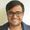Nov 29, 2021 2 min read

# How to Compare Strings in Bash Shell Scripting

In this tutorial you'll learn how to compare strings in bash shell scripts.You'll also learn to check if a string is empty or null.

Dealing with strings is part of any programming language. Bash shell scripting is no different. Even the syntax is pretty much the same.

In this quick tutorial, I’ll show you how to compare strings in Bash shell scrips.

## Bash string comparison

Here is how you compare strings in Bash.

``if [ "\$string1" == "\$string2" ]``

You can also use a string directly instead of using a variable.

``if [ "\$string1" == "This is my string" ]``

Let me show it to you with proper examples.

### Check if two strings are equal

If you want to check if two strings are equal, here’s an example:

``````#!/bin/bash
string1="MyString"
string2="MyString"
if [ "\$string1" == "\$string2" ]
then
echo "Equal Strings"
else
echo "Strings not equal"
fi``````

#### Tip: Pay Attention on the spaces

There must be a space between the `[` and the variable name and the equality operator ==. If you miss any of the spaces here, you’ll see an error like ‘unary operator expected’ or missing `]’.

### Check if strings are not equal in Bash

Instead of checking the quality, let’s do the opposite and check the inequality. Bash also provides the negation operator so that you can easily use “if not equal” condition in shell scripts.

``if [ "\$string1" != "Not MyString" ]``

The complete example looks like this:

``````#!/bin/bash
string1="MyString"

if [ "\$string1" != "Not MyString" ]
then
echo "Not Equal Strings"
else
echo "Strings equal"
fi``````

### Check if string is null or empty in Bash

Unlike some other languages like C++, in Bash you can check whether the string is null or empty with one single command:

``if [ -z "\$VAR" ]``

The -z actually checks if length of the variable is zero or not. If the variable is not set or if it is empty (equal to “”), the length will be zero and hence the condition will return true.

A complete example can be seen here:

``````#!/bin/bash
string1=
string2=""
if [ -z "\$string1" ]
then
echo "Null Strings"
fi
if [ -z "\$string2"]
then
echo "Empty String"
fi``````

Note on the use of single bracket ‘[]’ and double bracket ‘[[]]’ in bash scripts

You can also use the if statement with double brackets like this:

``if [[ "\$string1" == "My String" ]]``

The single bracket is the old Posix convention and it has some shortcomings. If you don’t use the double quotes around the variables and the variable is undefined, it will disappear from the code and this will result in syntax error.

``if [ \$string1 == "My String" ]``

If the variable \$string1 is empty or not defined in the above code, that line will become equivalent to

``if [  == "\$string2" ]``

Conclusion

I hope this quick little tutorial helped you in comparing strings in bash shell scripts. I recommend reading another quick tutorial on bash sleep command as well.